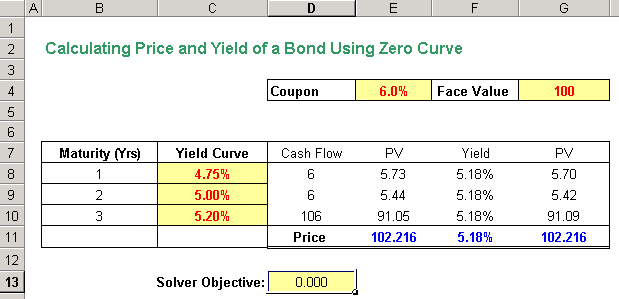Fixed Income

## Calculating Price and Yield of a Bond Using Zero Curve

In this post, we will demonstrate how you can calculate the price and the yield of a bond using a zero curve.

Assume that it is a 3-year bond that pays a coupon of 6% and has a \$100 par value. We also have the 3-year zero curve as shown below: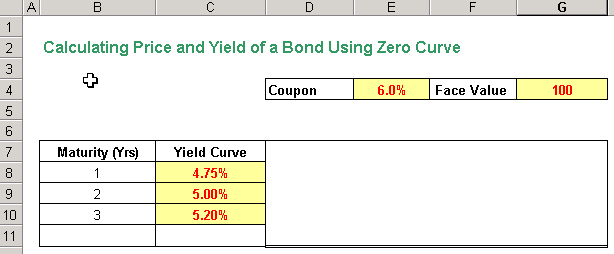The cash flows for this bond will be 6, 6, and 106 for the three years. The price of the bond will simply be the sum of the present value of the cash flows for these three years. The price of the bond is 102.216 as shown below. You can see that the price of the bond is above par. This is because the zero curve rates are below the coupon. If the coupon was lesser than the yield curve, the price would be less than par.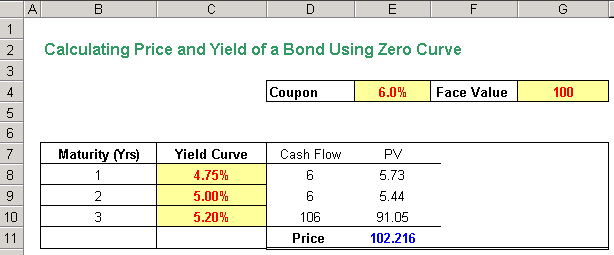We now need to calculate the yield of the bond. The yield of the bond will be the single rate, that, when used to calculate the price of the bond instead of the changing yield curve, will give the same price of the bond.

Assume that the yield is 7%, we can calculate the price of the bond by discounting each cash flow by 7% to get the price of the bond as shown below. The price of the bond this way is 97.376.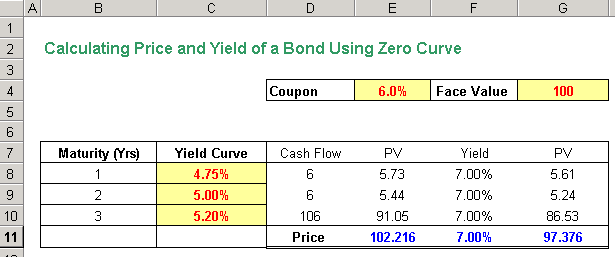However, this is not that same as 102.216, the actual price of the bond. So, we need to adjust the yield such that the price calculated using this rate is exactly 102.216. This can be done using solver. The objective of the solver is to get the yield in Cell F11 such that the difference between the two prices calculated (E11 – G11) is zero. This objective is set in cell D13.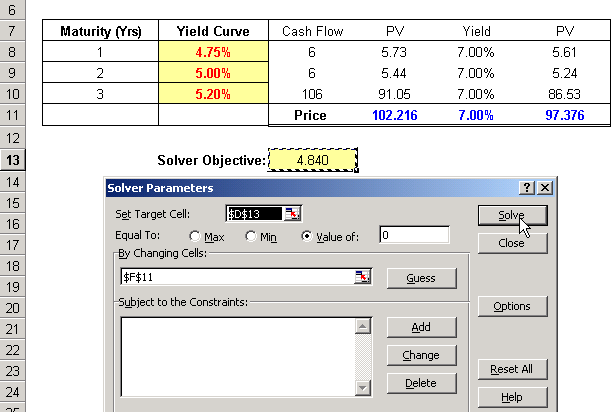Once you hit the solve button, the solver will solve it and get you the correct yield, which in this case is 5.18%.